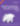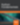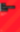# Operators in C

Programming

Share:

#### Free JavaScript Book!Write powerful, clean and maintainable JavaScript.

RRP \$11.95

Continuing on from my last article on the fundamentals of C, we’re now going to look at operators.

An expression is a sequence of operators and operands that computes a value. An operator in C is a symbol that tells the computer to perform mathematical or logical manipulation on data. The data items that operators act upon are called operands.

The different operators supported by C are:

1. Postfix Operators

2. Unary Operators

3. Cast Operators

4. Multiplicative Operators

6. Bitwise Shift Operators

7. Relational Operators

8.Equality Operators

9.Bitwise AND Operator

10.Bitwise Exclusive OR Operator

11.Bitwise Inclusive OR Operator

12. Logical AND Operator

13. Logical OR Operator

14.Conditional Operator

15. Assignment Operators

16. Comma Operators

## Postfix OperatorsMake the leap into server-side programming with a comprehensive cover of PHP & MySQL.

Normally RRP \$11.95 Yours absolutely free

An operator is called a Postfix Operator when the operator follows the operand. Postfix Operators may operate on either one or two operators. There are five postfix operators in C:-

1. Array subscripts

2. Function Call

3. Structure and Union Members

4. Postfix Increment and Decrement Operators

5. Compound Literals

### 1. Array Subscripts

We have already defined arrays in the previous article as  an identifier that refers to a collection of data items  that have the same name and the same data type. Each data item is represented  by their array element. The individual array elements are distinguished from one another by their  subscripts.

A postfix expression (operand followed by the operator) followed by an expression in square brackets [ ] is a subscripted designation  of the array element .The definition of the array subscript operator [ ]  is that ifis an array and is an  integer then` a[i] =*(a+i)`.

If the array subscripting operator [ ] is applied to an n dimensional  array x with dimensions n>=2  then `x` is converted to a pointer to an (n-1) dimensional array. Individual array elements are accessed by applying the indirection operator *.

To understand the above definition , the concept of pointers and the relation between arrays and pointers needs to be understood.

The name of the array always stores the address of the first element of the array. For example, if `x `is an integer array then `x `stores the address of the first element` x`. As `x` is an integer type array each element of the array will occupy 2 bytes. Therefore every element of an array can be accessed in terms of the offset added to the address of the first element. Here the offset is the subscript * memory occupied by the data type of the array. Therfore the second element `x `will be located at an offset of 2 bytes from the first element, the third element `x `at an offset of 4 bytes from the first element.

A pointer is a variable that stores the address of another variable. In these terms the array is referred to as a self-referential pointer as it stores its own address, i.e the address of the first element. Every element of an array  can therefore be represented in terms of a pointer (the array name) and an offset (the subscript). So `x `can be referred to as `x+1`, `x `which can be referred to as `x+2 `and so on. The value of the subscript determines the number of times the memory requirements must be added to the initial address to get the location of the subscripted element. This means that for the integer array `x`, one needs to find the address of the third element `x` and add the memory requirements (2 bytes)  twice to the initial address. Similarly the address of  `x` will be found by adding  2 bytes thrice to the initial address.

To find the actual value of an array element one needs to use the indirection operator *.  Therefore if `x+2 `denotes the memory address of the third element, `*(x+2) `will denote the value of the second element i.e the actual value of the second element stored in the array.

If a one-dimensional array can be represented in terms of a pointer(array name ) and an offset(subscript), multi-dimensional arrays can also be represented in similar terms. Therefore a two-dimensional integer array `x  `is actually a collection of two one-dimensional arrays each containing three data items. This two-dimensional array can be defined as a pointer to a group of one-dimensional arrays, where `*x` stores the address of (ie it points to) the first array of 3 elements, `*(x+1)` stores the address of the second array of three elements and so on. As each row is a one-dimensional array, `*(x)` stores the address of the first element of the first row, and` *(x+1)` stores the address of the first element of the second row.

In case of a multi-dimensional arrays with more than two dimensions` *(x)` or `*(x+1)` would not be storing the address of the first element of  each row. Rather they would  each be storing the initial address of multi-dimensional arrays. More about this when we discuss arrays and pointers in greater detail in a later article.

In an array `x[i][j]` , `x[i]`  is equal to `*(x+i)`, x is first converted to a pointer storing the inital address of the  first array of ` j` elements, `*(x+1)` stores the initial address of the second array, and so on. Now, the value of` i` defines the offset which by definition is the memory requirements’ * subscript value. The memory requirements here are the size of the  array of` j` elements. Considering our example of `x`, the memory requirements are the size of an individual array containing three integers each. If `*x` points to the initial address of the first array of three integers, `*(x+1)`will store the initial address of the second array of three integers which will be located at 3*2=6 bytes from the initial address of the first array. Thus it follows that multi- dimensional arrays are stored in a row major order.

For example: let `x= {{5,6,8}, {2,4,7}}`

then `* x `stores the initial address of the first row, ie of  {5,6,8}

`*(x+1)` stores the initial address of the second row {2,4,7}

Now each row will occupy 6 bytes, so if the initial address of `*x `(ie the address of the first row) is 1182

then the address of the second row  is 1182+6=1188; ie *(x+1) = 1188

Now to access the individual elements apply the indirection operator, for example: the second element of the first row will be located at `(*(x+0)+1)`  =1182+1*2 bytes = 1184

`*(x+0)` stores the address  of the first element of first row then `*(x+0)= x=5`

as the subscript gives the offset, then `*(x+0)= *(*(x+0)+0)=x=5`

Therefore,  `*(*(x+0)+0)`will give the first the first element of the first row.

Similarly `x =*(*(x+0)+1*2bytes))=6`

An array and a pointer are equivalent in many ways. Here, we’re looking at the basic concepts of arrays and pointers , so as to understand the array subscript operator. The array subscript operator will become completely clear once we discuss arrays and then pointers in detail.

### 2. Function Calls

In my earlier post Introduction to C, I gave an example of a function and introduced the concept of function prototype, function heading, return type and arguments. It would be helpful to recollect these concepts to understand the function call operator ( ).

A postfix expression followed by parentheses containing comma-separated arguments or no arguments at all is called a function call. The postfix expression denotes the called function.

The return type of a function may be  a function, a pointer, or any of the datatypes discussed earlier. If the return type of a function is a pointer, then the function returns an address.

A function argument is an expression that is used within the parentheses of a function call. A function parameter is an object declared within the parentheses of a function declaration or definition. When you call a function, the arguments are evaluated, and each parameter is initialized with the value of the corresponding argument, in the order in which they are present in the function call.

Type Conversions of Arguments

Arguments that are arrays or functions are converted to pointers before being passed as function arguments.

Arguments passed to non prototyped C functions undergo conversions: type  `char` parameters are converted to `int`, and `float` parameters to `double`. These are called default argument promotions.

The compiler compares the datatypes provided by the calling function with the datatypes that the called function expects and performs necessary type conversions, so if the called function does not include a prototype the arguments of the function are implicitly converted to the types of the function parameters.

If the function does not have a prototype, no other arguments  types are implicitly converted apart from those mentioned above.The number and type of arguments are not compared with the parameters of the called function if it does not have a function prototype declarator.

If the function is defined with a return type that is not compatible with the actual value being returned, you may get an error or undefined program behavior. For example, `char` and `int `are compatible, but `char` and `float` are not compatible. If the return type of a function is declared to be `char` but it returns a float, the program will give an error.

To understand the above points consider the following example:

```/*program to calculate the area of a circle using a user-defined function*/

#include <stdio.h>

#define PI 3.14159

int main()

{

printf("Area =%f", area);

return 0;

}

float process( float r)// function  expects a float argument

{

float a; /*local variable declaration*/

a= PI*r*r;
return(a); //function returns value.

}```

If in the above program the function prototype was missing , and if the function expected a value of type `double` and the  value of radius passed was of type `float`, the value of radius being passed would implicitly have been converted to type `double`.

Also if the function was declared to be of  type `void` and you would be returning a value through the return statement, there would have been a type compatibility error.

There is much more to understanding the working of functions, but we’ll discuss them in a future article on functions. Watch out for an article to be published shortly which will continue with investigating operators in C.### Surabhi Saxena

My name is Surabhi Saxena, I am a graduate in computer applications. I have been a college topper, and have been awarded during college by the education minister for excellence in academics. I love programming and Linux. I have a blog on Linux at www.myblogonunix.wordpress.com.

#### New books out now!Learn valuable skills with a practical introduction to Python programming!Give yourself more options and write higher quality CSS with CSS Optimization Basics.